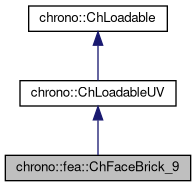chrono::fea::ChFaceBrick_9 Class Reference

## Description

Face of a linear ChElementBrick_9 tetrahedron.

This is a proxy to the tetrahedron. It can be used to apply pressure loads. Note, face_id is the number of the vertex to whom it is opposed: 0,1,2,3. Corner nodes, obtainable with GetNodeN(), are in counterclockwise order seen from the outside.

#include <ChFaceBrick_9.h>

Inheritance diagram for chrono::fea::ChFaceBrick_9:[legend]
Collaboration diagram for chrono::fea::ChFaceBrick_9:[legend]

## Public Member Functions

ChFaceBrick_9 (std::shared_ptr< ChElementBrick_9 > mel, char mid)

std::shared_ptr< ChNodeFEAxyzGetNodeN (int i)

void ShapeFunctions (ChVectorN< double, 4 > &N, double x, double y)
Fills the N shape function vector (size 4)

Gets the number of DOFs affected by this element (position part)

Gets the number of DOFs affected by this element (speed part)

virtual void LoadableGetStateBlock_x (int block_offset, ChState &mD) override
Gets all the DOFs packed in a single vector (position part)

virtual void LoadableGetStateBlock_w (int block_offset, ChStateDelta &mD) override
Gets all the DOFs packed in a single vector (speed part)

virtual void LoadableStateIncrement (const unsigned int off_x, ChState &x_new, const ChState &x, const unsigned int off_v, const ChStateDelta &Dv) override
Increment all DOFs using a delta.

virtual int Get_field_ncoords () override
Number of coordinates in the interpolated field: here the {x,y,z} displacement.

virtual int GetSubBlocks () override
Tell the number of DOFs blocks (ex. =1 for a body, =4 for a tetrahedron, etc.)

virtual unsigned int GetSubBlockOffset (int nblock) override
Get the offset of the i-th sub-block of DOFs in global vector.

virtual unsigned int GetSubBlockSize (int nblock) override
Get the size of the i-th sub-block of DOFs in global vector.

virtual void LoadableGetVariables (std::vector< ChVariables * > &mvars) override
Get the pointers to the contained ChVariables, appending to the mvars vector.

virtual void ComputeNF (const double U, const double V, ChVectorDynamic<> &Qi, double &detJ, const ChVectorDynamic<> &F, ChVectorDynamic<> *state_x, ChVectorDynamic<> *state_w) override
Evaluate N'*F , where N is some type of shape function evaluated at U,V coordinates of the surface, each ranging in -1..+1 F is a load, N'*F is the resulting generalized load Returns also det[J] with J=[dx/du,..], that might be useful in gauss quadrature. More...

virtual bool IsTriangleIntegrationNeeded () override
If true, use quadrature over u,v in [0..1] range as triangle volumetric coords Regular quadrature used for this element.

virtual ChVector ComputeNormal (const double U, const double V) override
Gets the normal to the surface at the parametric coordinate u,v. More...

## Protected Attributes

char face_id

std::shared_ptr< ChElementBrick_9melement

## ◆ ComputeNF()

 virtual void chrono::fea::ChFaceBrick_9::ComputeNF ( const double U, const double V, ChVectorDynamic<> & Qi, double & detJ, const ChVectorDynamic<> & F, ChVectorDynamic<> * state_x, ChVectorDynamic<> * state_w )
inlineoverridevirtual

Evaluate N'*F , where N is some type of shape function evaluated at U,V coordinates of the surface, each ranging in -1..+1 F is a load, N'*F is the resulting generalized load Returns also det[J] with J=[dx/du,..], that might be useful in gauss quadrature.

Parameters
 U parametric coordinate in surface V parametric coordinate in surface Qi Return result of N'*F here, maybe with offset block_offset detJ Return det[J] here F Input F vector, size is = n.field coords. state_x if != 0, update state (pos. part) to this, then evaluate Q state_w if != 0, update state (speed part) to this, then evaluate Q

## ◆ ComputeNormal()

 virtual ChVector chrono::fea::ChFaceBrick_9::ComputeNormal ( const double U, const double V )
inlineoverridevirtual

Gets the normal to the surface at the parametric coordinate u,v.

Normal must be considered pointing outside in case the surface is a boundary to a volume.